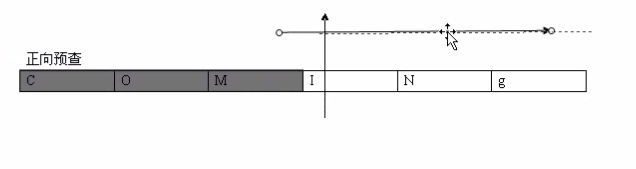# JavaScript 正则应用详解【模式、欲查、反向引用等】

更新时间：2020年05月13日 10:09:01   作者：alogy

## 正则应用

...

reg.test(str); 返回true 或者false 。 常在表单验证中使用。

```<from action="xxx.php" id="biaodan">
<p>请输入姓名：<input type="text" id="name" /></p>
<p>请输入年龄：<input type="text" id="age" /></p>
<p><input type="submit" /></p>
</from>
<script>
var oBD = document.getElementById('biaodan');
var oName = document.getElementById('name');
var oAge = document.getElementById('age');
//表单试图提交的时候，触发onsubmit事件
//这个函数返回了false,表单不会被提交
oBD.onclick = function(){
//验证name
if( !/^[\u4e00-\u9fa5]{2,4}\$/.test(oName.value) ) return false;
//验证年龄
if( !/^\d{2,3}\$/.test(oAge.value) ) return false;
if( parseInt( oAge.value )<10 || parseInt( oAge.value )>104 ) alert('您输入的年龄不在范围') return
false;
return true;
}
</script>
```

exec(); 返回 数组 或 null。
exec是英语execute的意思，CEO首席执行官，E就是executive执行的
“执行” 把正则式放到字符串上执行

```var str = 'ABCDEFG1234567abcdefg';
var reg = /[a-z]/g;
console.log( a=/[a-z]/g.exec(str) );
var a;
while( a=reg.exec(str) ){ //这边 null 为 fasle。 exec() 会保持对前面一次的引用。 需要使用 值来赋值。
console.log( a );
}
```

```var str = 'AAABBBCCCCCCC';
var reg = /(\w)\1+/g;
var maxLength = 0;
var maxLetter = '';
var a;
while( a=reg.exec(str) ){
if( a.length>maxLength ){
maxLength = a.length;
maxLetter = a;
}
}
console.log( maxLetter );
``````var str='BCDEFG1234567abcdefg';
var reg = /[a-z]/g;
var a;
while( (a=reg.exec(str)) != null ){ //先赋值给a，然后再与后边判断。
console.log( a );
}
```str.match( reg ); //查找，匹配到，返回数组
str.split( reg ); //拆分，返回数组
str.serch( reg ); //查找位置
str.replace( reg,'new str'); //正则替换，返回string

```
//测试是否含有hi
var reg = /hi/; //仅看字符串是否有 hi
console.log( reg.test('hello') ); //fasle
console.log( reg.test('this is iqianduan') ); //true
//测试单词 hi
var reg01 = /\bhi\b/;
console.log( reg01.test('this is') ); //false
console.log( reg01.test('this is, hi,his') );//true
```

## 正则表达式 3 句话

### 要找什么字符

```//找不吉利的数字
//3,4,7
var reg = /[3,4,7]/; //字符集合， 不能使用 /347/ 字面值表示，是表示整体。
console.log( reg.test('12121212') );//false
console.log( reg.test('12341234') ); //true
```

```// var reg = //;
var reg = /[0-9]/;
console.log( reg.test('123afsdf') ); //true
console.log( reg.test('asdf') ); //false
//是否有大写字母
var reg = /[A-Z]/;
console.log( reg.test('asdf') );//false
console.log( reg.test('Tomorrow is another day') ); //true
```

• `[0-9]` --> `\d`

• `[0-9a-zA-Z_]` --> `\w` .. 域名，注册用户名常用的模式.

• `[\t\v\f\r\n]` --> `\s` 空白符.

```//是否含有数字
var reg = /\d/;
console.log( reg.test('123afsdf') ); //true
console.log( reg.test('asdf') ); //false
```

• `[0-9]`---> `[^0-9]` ^ 脱字符号： 念法： caret。['kærət] 。

• `[abcdef]`-->`[^abcdef]`

//验证全为数字
var reg = /^[0-9]/; //匹配非数字
// var reg = /^d/ //字符簇补集
console.log( reg.test('aaaaa') );//非数字存在 false
console.log( reg.test('123aaa') ); //有数字存在 true

• d -- > D(非数字)

• s --> S(非空白字符)

• w --> W

• 任意字符 ： . 唯独不包括换行符

### 从哪儿找，找到哪儿

b 单词边界

/bhi/ --> 从单词的边界开始匹配hi。

```// 匹配单词hi,包括hi本身
// var reg = /\bhi.+/;//错误
// var reg = /\bhi\w+/; //错误。 + --> 至少有 一个
var reg = /\bhi\w*/;
console.log( reg.exec('this is') ); //null
console.log( reg.exec('his') ); //["his", index: 0, input: "his"]
console.log( reg.exec('history') ); //["history", index: 0, input: "history,hi"]
```

```//匹配进行时的结尾
var reg = /\b[a-zA-Z]+ing\b/;
console.log( reg.exec('going') );//["going", index: 0, input: "going"]
console.log( reg.exec('1ting.com') );//null
console.log( reg.exec('ing') );//null //2 -> to 4->for 0->zero
```

```//匹配un前缀的反义词
//unhappy happy,hungry,sun,unhappy
var reg = /\bun[\w]+\b/;
console.log( reg.exec('happy,hungry,sun,unhappy') ); //["unhappy", index: 17, input: "happy,hungry,sun
,unhappy"]
```

B 单词的非边界

```// 把单词中间的某一个部分取出来。
// 把中间含有hi的单词取出，即hi不能在两端。
var reg = /\Bhi\B/;
console.log( reg.exec('this') ); //["hi", index: 1, input: "this"]
console.log( reg.exec('hi') ); //null
```

^ creat , 从字符串的起始位置开始匹配

\$ 匹配到字符串的结束位置

```var reg = /^lishi\$/;
console.log( reg.exec('lishinihao') ); null
console.log( reg.exec('lishi') ); //["lisi", index: 0, input: "lisi"]
```

### 找多少

`*`， [0,n] --> {0, }
`+` , [1,n] -->{1, }
? , [0,1] -->{0,1}
n {n} {3} a{n} , 字符a准确的出现n次
a{n,} 字符a，至少出现n次。
a{n,m} 字符串a，出现n到m次。

### 模式

g -> 全局模式， global 找所有的，而不是找一次就结束
i -> 忽略大小写，ignore

```//查找所有中间含有hi的单词
var reg = /\Bhi\B/gi;
var str = 'shit,hi,this,thit,THIS';
console.log( str.match(reg) ); //["hi", "hi", "hi", "HI"]
```

```//把链接换成 #
//<a href="http://www.baidu.com" rel="external nofollow" rel="external nofollow" rel="external nofollow" rel="external nofollow" rel="external nofollow" rel="external nofollow" rel="external nofollow" ></a> --> <a href="#" rel="external nofollow" rel="external nofollow" rel="external nofollow" rel="external nofollow" rel="external nofollow" ></a>
//1,不能保留链接的文字(反向引用)
//2,不能跨行(贪婪模式)
var reg = /<a[\s]+.*<\/a>/g;
var str = '<a href="http://www.baidu.com" rel="external nofollow" rel="external nofollow" rel="external nofollow" rel="external nofollow" rel="external nofollow" rel="external nofollow" rel="external nofollow" ></a>';
console.log( str.replace(reg,'<a href="#" rel="external nofollow" rel="external nofollow" rel="external nofollow" rel="external nofollow" rel="external nofollow" >文字</a>') );
```

js不支持单行模式。

```//s 单行模式：把整个字符串看成一行

. 代表任意，但不包括换行。

```

sS 全部字符 使用一个技巧， 一个集合加补集，就是全集
[dD] [sS] [wW]

```var reg = /\<a[\s][\s\S]+<\/a>/g;
var str = '<a href="http://www.baidu.com" rel="external nofollow" rel="external nofollow" rel="external nofollow" rel="external nofollow" rel="external nofollow" rel="external nofollow" rel="external nofollow" >'+
'</a>';
console.log( str.replace(reg,'<a href="#" rel="external nofollow" rel="external nofollow" rel="external nofollow" rel="external nofollow" rel="external nofollow" >文字</a>') );

//s 多行模式：碰到一行就把当前的当成一个字符串来解析
```
```//把每一行的结尾的数字换成 #
//车牌号
//Cx003
//A0008
//B3456
var str = 'Cx003'+
'A0008'+
'B3456';
var reg = /\d+\$/gm;
console.log( str.replace(reg,'#') );
```

## 贪婪模式

```//goooooooooods --> goods
var str = 'goooooooooods,goooods,goooood,gooooo,gooooods';
var reg = /g[o]{3,}?ds/g;
console.log( str.replace(reg,'goods') ); //goods,goods,goooood,gooooo,goods

```

## 欲查

### 正向欲查

```//查找进行时的单词的词根， 即 不要ing 。 going -> go
var str = 'going,comming,fly';
// var reg = /\b[a-zA-Z]+ing\b/g;
var reg = /\b[\w]+(?=ing)\b/g; // 类似探照灯，先去判断几位是否满足，满足返回，不满足继续下一位.
console.log( str.match(reg) );
``````//查找进行时的单词的词根， 即 不要ing 。 going -> go
var str = 'going,comming,fly';
// var reg = /\b[a-zA-Z]+ing\b/g;
// var reg = /\b[a-zA-Z]+(?=ing)\b/g; //结尾\b 是错误的， 欲查不消耗字符， 相当于/\b[a-zA-Z]+\b/ 这种形式
var reg = /\b[a-zA-Z]+(?=ing)/g; // 类似探照灯，先去判断几位是否满足，满足返回，不满足继续下一位.
console.log( str.match(reg) ); // ["go", "comm"]

```

### 负向欲查```//查找win98,win95,win32,win2003,winxp -->win98,win32,win2003,winxp
var str = 'win98,win95,win32,win2003,winxp';
var reg = /\bwin(?!95)/g;
console.log( str.match(reg) ); // ["win", "win", "win", "win"]

```

js不支持，向前正向欲查，向前负向欲查：

```//找出 un系列单词的词根
var reg = /[\w]+(?<=un)/g;
var str = 'unhappy';
console.log(str.match(reg)); //报错
var reg = /[\w]+(?<!=un)/g; //向前负向欲查
```

## 反向引用

```//把链接换成空连接，保持文字信息。
var str = '<a href="http://www.baidu.com" rel="external nofollow" rel="external nofollow" rel="external nofollow" rel="external nofollow" rel="external nofollow" rel="external nofollow" rel="external nofollow" >阴天快乐</a>';
var reg = /<a[\s]+[^>]+>([^<>]+)<\/a>/; //超链接的表达式
console.log( reg.exec(str) ); //["<a href="http://www.baidu.com" rel="external nofollow" rel="external nofollow" rel="external nofollow" rel="external nofollow" rel="external nofollow" rel="external nofollow" rel="external nofollow" >阴天快乐</a>", "阴天快乐", index: 0
, input: "<a href="http://www.baidu.com" rel="external nofollow" rel="external nofollow" rel="external nofollow" rel="external nofollow" rel="external nofollow" rel="external nofollow" rel="external nofollow" >阴天快乐</a>"] //能够把子表达式的东西匹配出来。
// console.log( str.replacte(reg,'#') );
/**
<a[\s]+[^>]>([^<>]+)<\/a> 主要目的是想要中间那一块
除了>之外的都可行 ， 取> 的补集 [^>]
中间部分纯文字，不含大于号，和小于号。 取小于号和大于号的补集 [^<>]+ / [\s\S]+
*/
//一般是整个表达式， 但是中间的部分 有特殊做了描述。 需要的部分特殊处理。使用分组，叫做子表达式。
//匹配html
// /<\s*(\S+)(\s[^>]*)?>[\s\S]*<\s*\/\1\s*>/
/*exec为例：
匹配到的数组，第0个单元，代表"整个正则表达式的匹配结果"
1,2,3,4....N,则代表第N个子表达式匹配的结果。 //js顶多有9个子表达式。 // ["<a href="http://www.baidu
.com">阴天快乐</a>", "阴天快乐", index: 0, input: "<a href="http://www.baidu.com" rel="external nofollow" rel="external nofollow" rel="external nofollow" rel="external nofollow" rel="external nofollow" rel="external nofollow" rel="external nofollow" >阴天快乐</a>"]
*/
console.log( str.replace(reg,'<a href="#" rel="external nofollow" rel="external nofollow" rel="external nofollow" rel="external nofollow" rel="external nofollow" >\$1</a>') ); //<a href="#" rel="external nofollow" rel="external nofollow" rel="external nofollow" rel="external nofollow" rel="external nofollow" >阴天快乐</a>

var str = '<html></html>';
var reg = /<\s*(\S+)(\s[^>]*)?>[\s\S]*<\s*\/\1\s*>/;
console.log( reg.exec(str) );
str.replace(reg,function( \$1,\$2 ){
console.dirxml(\$2); //html
});
```

• 在正则外边使用：\$N 来匹配 第N个子表达式的匹配结果。

• 在正则里边使用N来 使用第N个子表达式。